0
586

# Blood Relation Questions for IBPS-SO PDF

Download Important Blood Relation PDF based on previously asked questions in IBPS-SO and other Banking Exams. Blood Relation for IBPS-SO Exam.

Instructions

Study the information carefully and answer the questions that follow:
Lavkush is the husband of Devi. Lavkush has 3 children. Navin is the brother of Chang and Praneet. Praneet is the daughter of Lavkush.

Question 1: How is Devi related to Chang?

a) Sister

b) Daughter

c) Granddaughter

d) Mother

e) Can’t be determined

Instructions

If A+B means “A is the husband of B”
A-B means “A is the sister of B”
A*B means “A is the brother of B”
A\$B means “A is the father of B”

Question 2: If A+B-C*D is true, how is A related to D?

a) Cousin

b) Father

c) Brother

d) Brother-in-law

e) none of these

Question 3: Pointing towards a senior citizen, Ravi said, “This is my niece’s paternal grandmother’s sister-in-law.” How is Ravi related to the senior citizen?

a) brother

b) nephew

c) grandson

d) son-in-law

e) None of the above

Question 4: How is Ram’s mother’s niece’s father related to Ram?

a) Son

b) nephew

c) Cousin

d) Uncle.

e) Grandson

Instructions

Study the following information carefully and answer the questions
given below :

R is married to U. U is the mother of L. L is the sister of D. U has only one daughter. D is married to J. K is the son of J. F is the mother of J.

Question 5: How is D related to F ?

a) Cannot be determined

b) Daughter

c) Daughter-in-law

d) Son-in-law

e) Son

Question 6: How is R related to K ?

a) Cannot be determined

b) Grandfather

c) Grandmother

d) Father

e) Uncle

Instructions

Study the following information carefully and answer the questions given below :
K is the brother of J. J has only one daughter. J is the mother of L. L is the sister of T. B is the father of T. T is married to R.

Question 7: How is J related to R ?

a) Cannot be determined

b) Sister-in-law

c) Mother

d) Aunt

e) Mother-in-law

Question 8: How is T related to K ?

a) Niece

b) Cannot be determined

c) Nephew

d) Son

e) Daughter

Instructions

Study the following information carefully and answer the questions given below :

Q is the sister of tr. T is the mother of D. T has only one son. D is the brother df J. J
is married to M. Y is the daughter of M.

Question 9: How is J related to Q ?

a) Son

b) Cannot be determined

c) Daughter

d) Niece

e) Nephew

Question 10: How is Y related to T ?

a) Daughter

b) Daughter-in-law

c) Cannot be determined

d) Niece

e) Grand daughter

Instructions

Study the following information and answer the given questions.
• D is daughter of N. E is wife of N.
• G is sister of D. C is married to G.
• N has no son. K is mother of E.
• Q is only daughter of C.

Question 11: How Q is related to D ?

a) Daughter

b) Cousin

c) Niece

d) Sister­in­law

e) Cannot be determined

Question 12: How N is related to K ?

a) Brother-­in-­law

b) Cousin

c) Son-­in-­law

d) Sister

e) Brother

Question 13: How many daughters N have ?

a) One

b) Three

c) Two

d) Cannot be determined

e) None of these

Instructions

Study the given information carefully to answer the given questions.

A is the mother of B. B is the sister of C. D is the son of C. E is the brother of D. F is the mother of E. G is the granddaughter of A. H has only two childrenB and C.

Question 14: How is F related to H ?

a) Son-in-law

b) Daughter-in-law

c) Father-in-law

d) Granddaughter

e) Niece

Question 15: How is C related to E ?

a) Father

b) Son

c) Mother

d) Cousin

e) Grandfather

Praneet is the daughter of Lavkush. Chang, Praneet and Navin are siblings. So, Chang, Naveen and Praneet are the children of Lavkush and Devi. So, Devi is Sam’s mother.

A+B-C*D ==> A is the husband of B, B is the sister of C, C is the brother of D.
==>A is the brother-in-law of D.
So correct option to choose is D.

Ravi’s niece’s paternal grandmother is Ravi’s sister-in-law’s mother. Her sister-in-law is Ravi’s sister-in-law’s aunt. So, Ravi is the nephew-in-law of the senior citizen.

Ram’s mother’s niece is Ram’s cousin. Her father is Ram’s uncle.

R is married to U, who is mother of L, => R is husband of U.

U has only one daughter and L is the sister of D, => D is brother of L.

D is married to J and has a son K.

The flow chart will be :

where, (m) represents male

(f) represents female

— represents married couple

= represents siblings

| represents children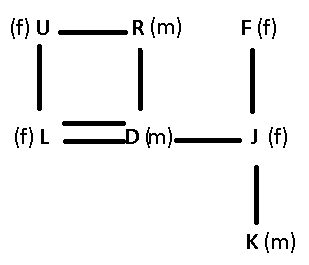D is the husband of F’s daughter, => D is son-in-law of F.

Ans – (D)

R is married to U, who is mother of L, => R is husband of U.

U has only one daughter and L is the sister of D, => D is brother of L.

D is married to J and has a son K.

The flow chart will be :

where, (m) represents male

(f) represents female

— represents married couple

= represents siblings

| represents childrenR is father of D, who is father of K, => R is grandfather of K.

Ans – (B)

J has only one daughter and is mother of L, who is sister of T, => T is brother of L(female).

=> T is son of B(male) and husband of R.

The flow chart will be :

where, (m) represents male

(f) represents female

— represents married couple

= represents siblings

| represents children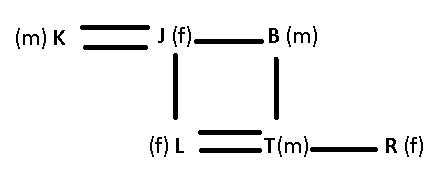J is the mother of R’s husband, => J is mother-in-law of R.

Ans – (E)

J has only one daughter and is mother of L, who is sister of T, => T is brother of L(female).

=> T is son of B(male) and husband of R.

The flow chart will be :

where, (m) represents male

(f) represents female

— represents married couple

= represents siblings

| represents childrenT is the son of K’s sister, => T is the nephew of K.

Ans – (C)

Q is the sister of T, who is mother of D. T has only 1 son while D is brother of J, => J is daughter of T.

J is married to M having a daughter, Y.

The flow chart will be :

where, (m) represents male

(f) represents female

— represents married couple

= represents siblings

| represents children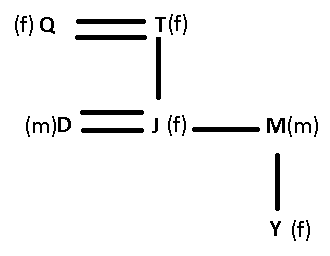J is the daughter of Q’s sister, => J is the niece of Q

Ans – (D)

Q is the sister of T, who is mother of D. T has only 1 son while D is brother of J, => J is daughter of T.

J is married to M having a daughter, Y.

The flow chart will be :

where, (m) represents male

(f) represents female

— represents married couple

= represents siblings

| represents childrenY is the daughter of J, who is daughter of T, => Y is grand daughter of T.

Ans – (E)

D is daughter of N, and sister of G, and E is wife of N, => N is husband of E, and they have two daughters D & G.

C is husband of G having one daughter Q. K is mother of E.

The flow chart will be :

where, (m) represents male

(f) represents female

— represents married couple

= represents siblings

| represents children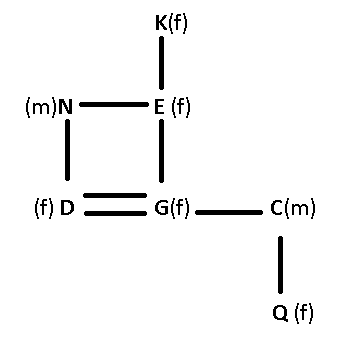Q is daughter of D’s sister, => Q is the niece of D.

Ans – (C)

D is daughter of N, and sister of G, and E is wife of N, => N is husband of E, and they have two daughters D & G.

C is husband of G having one daughter Q. K is mother of E.

The flow chart will be :

where, (m) represents male

(f) represents female

— represents married couple

= represents siblings

| represents childrenN is the husband of K’s daughter, => N is son-in-law of K.

Ans – (C)

D is daughter of N, and sister of G, and E is wife of N, => N is husband of E, and they have two daughters D & G.

C is husband of G having one daughter Q. K is mother of E.

The flow chart will be :

where, (m) represents male

(f) represents female

— represents married couple

= represents siblings

| represents childrenN has two daughters : D & G

Ans – (C)

A is the mother of B, who is the sister of C, and H has two children B & C, => A is the wife of H and they have two children B & C.

D is the son of C, and D and E are brothers, since F is the mother of E, => C is the husband of F, and they have two sons D & E.

The flow chart will be :

where, (m) represents male

(f) represents female

— represents married couple

= represents siblings

| represents children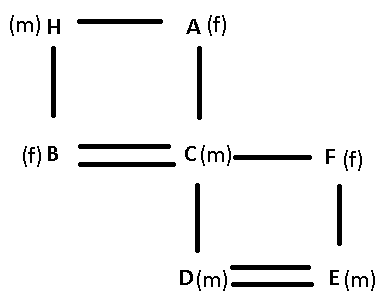F is the wife of H’s son, => F is daughter-in-law of H.

Ans – (B)

A is the mother of B, who is the sister of C, and H has two children B & C, => A is the wife of H and they have two children B & C.

D is the son of C, and D and E are brothers, since F is the mother of E, => C is the husband of F, and they have two sons D & E.

The flow chart will be :

where, (m) represents male

(f) represents female

— represents married couple

= represents siblings

| represents childrenClearly, C is the father of E.

Ans – (A)